Next: 6.4.2 Numerical Solution Techniques Up: 6.4 Vertical Discretization of Previous: 6.4 Vertical Discretization of

## 6.4.1 Two-Point Boundary Value Problem

The final problem can be stated as follows: The ODE system (6.34)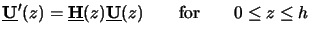(6.33) has to be solved subject to the BCs (6.51)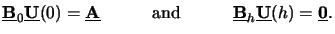(6.34)

Here we have changed the notation slightly to treat all excitation vectors apq as well as the different solutions upq(z) resulting therefrom simultaneously. The multiple upq(z) and apq are comprised into the two matrices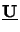(z) and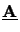, respectively, i.e,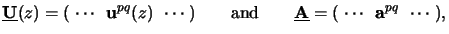(6.35)

whereas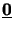refers to the zero-matrix and is of the same dimension as. The ODE, i.e., the system matrix(z) in (6.52), stays the same for all source point contributions (p, q). This stems from the fact that the source point locations are restricted to the special grid illustrated in Figure 4.8 which yields only periodic fields incident on the photoresist (c.f. (4.55)). To avoid any misunderstanding of the used notation we summarize the dimensions as well as the meaning of all quantities occurring in the final formulation (6.52) to (6.54) of the two-point BVP in Table 6.1.

Table 6.1: Dimension and meaning of the quantities used in the differential method. NODE denotes the number of ordinary differential equations, and Ns refers to the number of incident source point contributions.

 Quantity Dimension Meaning Equation(z) NODE x Ns Solution matrix (6.52), (6.54)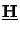(z) NODE x NODE System matrix (6.52)NODE/2 x Ns Upper excitation matrix (6.53), (6.54)NODE/2 x Ns Lower excitation matrix (6.53)NODE/2 x NODE Upper boundary matrix (6.53)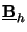NODE/2 x NODE Lower boundary matrix (6.53)

Special care has to be given to the multiple BCs. An algorithm that calculates all fundamental solutions of the ODE system independently of the BCs and then projects these general solutions to the BCs could treat the Ns BCs simultaneously and thus no extra costs would result. At a first glance this idea seems to be strange because calculating all solutions first and then filtering out only the required ones seems to be superfluous since a first-order NODE-dimensional ODE system has NODE fundamental solution vectors and Ns is always very small in comparison to NODE. However, exactly this idea can be implemented in a very tricky way to result in a fundamental advantage. Before we work out this strategy in Section 6.4.3 some general information about numerical techniques suited for BVPs is provided in the following section.Next: 6.4.2 Numerical Solution Techniques Up: 6.4 Vertical Discretization of Previous: 6.4 Vertical Discretization of
Heinrich Kirchauer, Institute for Microelectronics, TU Vienna
1998-04-17